# Test: Statistical Description Of Data - 2

## 40 Questions MCQ Test Quantitative Aptitude for CA CPT | Test: Statistical Description Of Data - 2

Description
This mock test of Test: Statistical Description Of Data - 2 for CA Foundation helps you for every CA Foundation entrance exam. This contains 40 Multiple Choice Questions for CA Foundation Test: Statistical Description Of Data - 2 (mcq) to study with solutions a complete question bank. The solved questions answers in this Test: Statistical Description Of Data - 2 quiz give you a good mix of easy questions and tough questions. CA Foundation students definitely take this Test: Statistical Description Of Data - 2 exercise for a better result in the exam. You can find other Test: Statistical Description Of Data - 2 extra questions, long questions & short questions for CA Foundation on EduRev as well by searching above.
QUESTION: 1

Solution:
QUESTION: 2

Solution:
QUESTION: 3

### The frequency distribution of a continuous variable is known as

Solution:
QUESTION: 4

The distribution of shares is an example of the frequency distribution of

Solution:
QUESTION: 5

The distribution of profits of a blue-chip company relates to

Solution:
QUESTION: 6

Analysis based on study of price fluctuations, production of commodities and deposits in banks is classified as

Solution:
QUESTION: 7

Mutually inclusive classification is usually meant for

Solution:
QUESTION: 8

Mutually exclusive classification is usually meant for

Solution:
QUESTION: 9

The LCB is

Solution:
QUESTION: 10

Branch of statistics which deals with development of particular statistical methods is classified as

Solution:
QUESTION: 11

length of a class is

Solution:
QUESTION: 12

For a particular class boundary, the less than cumulative frequency and more than cumulative frequency add up to

Solution:
QUESTION: 13

Frequency density corresponding to a class interval is the ratio of

Solution:
QUESTION: 14

Relative frequency for a particular class

Solution:
QUESTION: 15

​Mode of a distribution can be obtained from

Solution:
QUESTION: 16

Median of a distribution can be obtained from

Solution:
QUESTION: 17

A comparison among the class frequencies is possible only in

Solution:
QUESTION: 18

Frequency curve is a limiting form of

Solution:
QUESTION: 19

Most of the commonly used frequency curves are

Solution:
QUESTION: 20

The distribution of profits of a company follows

Solution:
QUESTION: 21

(Direction 21 - 27) Answer the following questions. Each question carries 2 marks.

Q. Out of 1000 persons, 25 per cent were industrial workers and the rest were agricultural workers. 300 persons enjoyed world cup matches on TV. 30 per cent of the people who had not watched world cup matches were industrial workers. What is the number of agricultural workers who had enjoyed world cup matches on TV?

Solution:
QUESTION: 22

A sample study of the people of an area revealed that total number of women were 40% and the percentage of coffee drinkers were 45 as a whole and the percentage of male coffee drinkers was 20. What was the percentage of female non-coffee drinkers?

Solution:
QUESTION: 23

Cost of sugar in a month under the heads Raw Materials, labour, direct production and others were 12, 20, 35 and 23 units respectively. What is the difference between the central angles for the largest and smallest components of the cost of sugar?

Solution:
QUESTION: 24

The number of accidents for seven days in a locality are given below :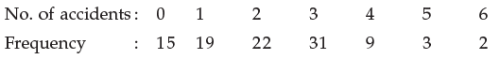Q. What is the number of cases when 3 or less accidents occurred?

Solution:
QUESTION: 25

The following data relate to the incomes of 86 persons :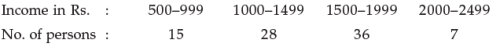Q. What is the percentage of persons earning more than Rs. 1500?

Solution:
QUESTION: 26

The following data relate to the marks of a group of students: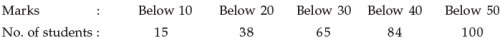Q. How many students got marks more than 30?

Solution:
QUESTION: 27

In which files, the records are organised in sequence and an index table is used to speed up access to the records without requiring a search of the entire file?

Solution:
QUESTION: 28

(Direction 28 - 32) Answer the following questions. Each question carries 5 marks.

In a study about the male and female students of commerce and science departments of a college in 5 years, the following datas were obtained :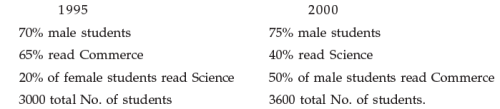Q. After combining 1995 and 2000 if x denotes the ratio of female commerce student to female Science student and y denotes the ratio of male commerce student to male Science student, then

Solution:

The correct option is Option A.

1995

70% male students = 2100 (70% of 3000)

30% female students = 900

20% of female read science = 180

80% of female read commerce = 720

Male students read commerce = 1230 (1950 - 720)

Male students read science = 870

2000

75% male students = 2700 (75% of 3600)

25% female students = 900

50% of male students read commerce = 1350

50% of male students read science = 1350

Female students of science = 90

Female students of commerce = 810

x = female commerce students / female science students

= 720 + 810 / 180 + 90

= 1530 / 270

= 5.666

y = male commerce students / female commerce students

= 1230 + 1350 / 870 + 1350

= 2580 / 2220

= 1.162

So, x > y

Hence proved

QUESTION: 29

In a study relating to the labourers of a jute mill in West Bengal, the following information was collected.

‘Twenty per cent of the total employees were females and forty per cent of them were married. Thirty female workers were not members of Trade Union. Compared to this, out of 600 male workers 500 were members of Trade Union and fifty per cent of the male workers were married. The unmarried non-member male employees were 60 which formed ten per cent of the total male employees. The unmarried non-members of the employees were 80’. On the basis of this information, the ratio of married male non-members to the married female non-members is

Solution:
QUESTION: 30

The weight of 50 students in pounds are given below :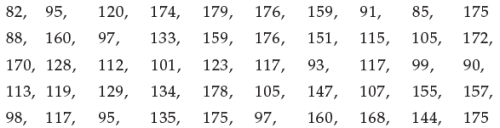Q. If the data are arranged in the form of a frequency distribution with class intervals as 81-100, 101-120, 121-140, 141-160 and 161-180, then the frequencies for these 5 class intervals are

Solution:
QUESTION: 31

The following data relate to the marks of 48 students in statistics :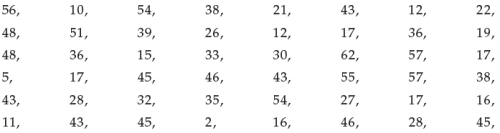Q. What are the frequency densities for the class intervals 30-39, 40-49 and 50-59

Solution:
QUESTION: 32

The following information relates to the age of death of 50 persons in an area :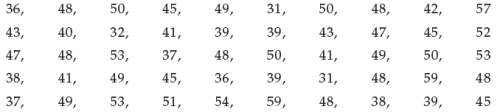Q. If the class intervals are 31-33, 34-36, 37-39, …. Then the percentage frequencies for the last five class intervals are

Solution: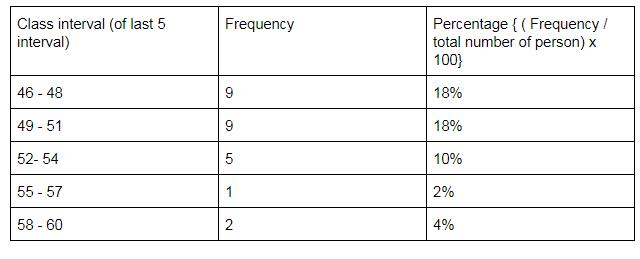QUESTION: 33

Graph is a

Solution:
QUESTION: 34

​Details are shown by

Solution:
QUESTION: 35

The relationship between two variables are shown in

Solution:
QUESTION: 36

In general the number of types of tabulation are

Solution:
QUESTION: 37

A table has

Solution:
QUESTION: 38

The number of errors in Statistics are

Solution:
QUESTION: 39

The number of “Frequency distribution“ is

Solution:
QUESTION: 40

(Class frequency)/(Width of the class ) is defined as

Solution: